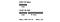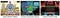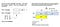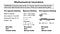# How do professional mathematicians solve problems?

Here is a simple-looking arithmetic puzzle you often come across in puzzle collections. How quickly can you solve it? (Normally I stress that mathematics is not about speed; indeed, most mathematicians I know work slowly, as do I. But this time it’s different. The goal is to see how you do when you work quickly.)

PROBLEM: A bat and a ball cost \$1.10. The bat costs \$1 more than the ball. How much does the ball cost on its own? (There is no special pricing deal.)

How did you do? The most common answer people give instantly to this problem is that the ball costs 10¢. That answer is wrong. But if that’s what you got, you are in good company. If anything, your answer likely shows that you are a good thinker with some well-developed problem-solving strategies. It just that in this case, the problem is worded to trip you up if you follow a strategy that usually works.

Here is, most likely, what your mind did to get to that 10¢ answer. As you read through the problem statement and came to that key phrase “cost more,” your mind said, “I will need to subtract.” You then took note of the data: those two figures \$1.10 and \$1. So, without hesitation, you subtracted \$1 from \$1.10 (the smaller from the larger, since you knew the answer has to be positive), getting 10¢.

Notice you did not really perform any calculation. The numbers are particularly simple ones. Almost certainly, you retrieved from memory the fact that if you take a dollar from a dollar-ten, you are left with 10¢. You might even have visualized those amounts of money in your hand.

What you did is apply a fast-thinking strategy — the technical term is a “heuristic” — you acquired over many financial transactions and very likely a substantial number of arithmetic quiz questions in elementary school. In fact, the timed tests in schools actively encourage such a “pattern recognition” approach. For the simple reason that it is fast and usually works!

Here is that heuristic: Identify the arithmetic operation involved and then plug in the data. It’s fast, requires no effort, and usually works. What makes it a smart approach is that it uses something the human brain is remarkably good at — pattern recognition — and avoids something our minds find difficult and requiring effort to master (namely, arithmetic calculation).

Another way you could have solved the problem was by applying an algorithm you had learned at school. Namely, you could have reasoned as follows:

Let x = cost of bat and y = cost of the ball. Then, we can translate the problem into symbolic form as: x + y = 1.10 , x = y + 1

Eliminate x from the two equations by algebra, to give: 1.10 — y = y + 1

Transform this by algebra to give: 0.10 = 2y

Thus, dividing both sides by 2, you conclude that: y = 5¢.

And this time, you get the correct answer.

You may, in fact, have been able to carry out this procedure in your head. When I was at school, I could do algebraic manipulations far more complicated than this in my head quickly, but truth be told, I long ago lost that skill, since I never used it outside the classroom.

Whether or not you can do the calculation in your head, it is of course entirely formulaic and routine. Unlike the first method I looked at (a heuristic that is fast and usually right), this method is an algorithmic procedure, it is slow (much slower than the first method, even when the algebraic reasoning is carried out in your head), but it always works.

It is also an approach that can be executed by a machine. True, for such a simple example, it’s quicker to do it by hand on the back of an envelope, but as a general rule, it makes no sense to waste the time of a human brain following an algorithmic procedure, not least because, even with simple examples it is familiarly easy to make a small error that leads to an incorrect answer.

But there is another way to solve the problem, that is typical of the ways professional mathematicians work. Like the first method I looked at, it is a heuristic, hence instinctive and fast, but unlike the first heuristic method, it always works.

This third method requires looking beyond the words, and beyond the symbols in the case of a problem presented symbolically, to the quantities represented. Though I (and likely other mathematicians) don’t visualize it quite this way (in my case it is more of a vague sense-of-size rather than neat rectangles), the figure below more or less captures what the pros do.Depiction of the mental image many professional mathematicians create in their mind to solve the bat-and-ball puzzle.

As we read the problem, we form a mental sense of the two quantities, the cost of the ball-on-its-own and the cost of the bat-plus-ball, together with the stated relation between them, namely that the latter is \$1 more than the former. From that mental image, where we see the \$1.10 total consists of three pieces, one of which has size \$1 and the other two of which are equal, we simply “read off” the fact that the ball costs 5¢. No calculation, no algorithm. Pure pattern recognition.

As I noted, exactly how professional mathematician visualize the problem likely varies. But when asked by a cognitive scientist to draw a picture of their reasoning, they almost always produce something like the drawing shown. We can, therefore, assume that the diagram gives us a valuable insight into the way professional mathematicians think. And the essence of that way of thinking is to go beyond the words and the symbols to some kind of picture of the numbers, quantities, or whatever that the problem is talking about, and then reason with that picture — usually by mentally manipulating it in their mind.The mathematical puzzles in the BrainQuake app are based on the kinds of mental images professional mathematicians create to solve problems. The puzzles are designed so that solving the puzzle on the screen requires following the same logical steps the pro does in their mind. Image by BrainQuake.

The kinds of mental pictures that the pros use provided the initial ideas for the puzzles we designed for the BrainQuake app. The idea was to Break the Symbol Barrier by taking players into the mind of the professional mathematician, so they could manipulate on a screen the image that the pro would manipulate in their mind. I should stress that such was the idea. It’s not exactly what is going on. We made the images clean, precise, and mechanical, and drew them in a way that makes for a fun puzzle-game experience, complete with sound effects. So when you solve one of our puzzles, you are not doing exactly what the pros do in their heads. But the logical reasoning sequence you must follow to solve the puzzle is closely aligned to what the pros do in their heads. (We design the puzzle so that this has to occur.) It doesn’t mean you can solve puzzles like a pro. But it takes you a good way on the path to learning how to do what they do.

Using illustrations of the mental images mathematicians create in their minds when solving problems is not unique to BrainQuake, or to learning games. The famous Singapore Mathematics instruction method uses such figures as depictions to help students manipulate formulas and equations. In their case, the diagrams, which are static, are aids to help the student form their own mental images as they reason with the symbols. In our case, we make the diagrams dynamic and interactive so the student can manipulate them directly, which offers some considerable advantage in the earlier stages of math learning.Singapore Mathematics uses (static) depictions of the mental images professional mathematicians create to solve problems, to provide visual support for the mathematical reasoning the student follows in symbolic form.

Incidentally, the Australian mathematician (and former child prodigy) Terrence Tao has called those three ways of solving the bat-and-ball problem, respectively, pre-rigorous thinking, rigorous thinking, and post-rigorous thinking. In the blog in which he gave those definitions, he was discussing the way professional mathematicians solve abstract problems in pure mathematics. The formal, symbolic, rigorous descriptions they lay out in their papers and books come primarily at the end of their work, when they need to check that the solution is logically correct, or at various intermediate points to make those checks along the way. But their key thinking is almost always a process of post-rigorous heuristics.The three kinds of solution to the bat-and-ball problem. The celebrated Australian mathematician Terrence Tao has called the three kind of thinking “pre-rigorous”, “rigorous”, and “post-rigorous”.

For the student learning mathematics, the ultimate educational goal is to develop a useful set of post-rigorous heuristics. That almost certainly requires achieving some level of master of rigorous thinking. (Willingham (2010) presents evidence to that effect.) Traditionally, that was achieved by starting with pre-rigorous thinking, even though that would ultimately be jettisoned. BrainQuake mathematics education provides a pathway towards mastery of rigorous thinking using post-rigorous heuristics that do not have to be thrown away later, but indeed provide a foretaste of the way the pros work. Our goal is not to replace your math teacher, rather to provide valuable assistance to help you do well in the algebra class (and hopefully beyond).

Here’s to a rich collection of heuristics!

– Keith